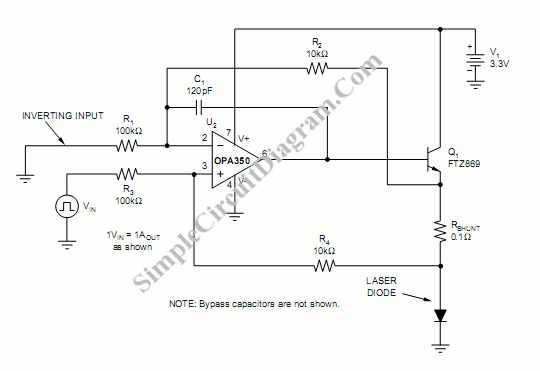# Laser Diode Driver

We can use a voltage-controlled current source to implement a laser diode driver. Compared with a a Switched (PWM) drivers, this simple  linear laser diode driver provides cleaner drive current. Here is the schematic diagram of the circuit:As the basic of this laser diode driver, this circuit uses a Howland current pump with a current booster. The output of a R-R CMOS OPA350 op amp (U2) uses Q1 as the current booster. To sense the current, this circuit uses voltage drop across a shunt resistor (RSHUNT) in series with the laser diode that is  measured differentially. Analog voltage of the microprocessor’s  potentiometer which is controlled by the DAC can be fed to the VIN to control the laser diode current. If we use the values shown in the figure, the scale factor of  output is 1V input equals 1A. Other values of scale factor (VIN/IOUT) can be set by choosing different resistor values using the equation:

VIN/IOUT=RSHUNT(R3/R4), with R1=R3, and R2=R4. [source: Burr-Brown Products Application Report]